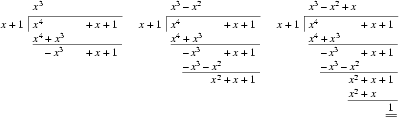Online JudgeProblem SetAuthorsOnline ContestsUser
Web Board
F.A.Qs
Statistical Charts
Problems
Submit Problem
Online Status
Prob.ID:
Register
Authors ranklist
Current Contest
Past Contests
Scheduled Contests
Award Contest
Register
Language:
Polynomial Remains
 Time Limit: 1000MS Memory Limit: 65536K Total Submissions: 1463 Accepted: 821

DescriptionGiven the polynomial
a(x) = an xn + ... + a1 x + a0,

compute the remainder r(x) when a(x) is divided by xk+1.

Input

The input consists of a number of cases. The first line of each case specifies the two integers n and k (0 <= n, k <= 10000). The next n+1 integers give the coefficients of a(x), starting from a0 and ending with an. The input is terminated if n = k = -1.

Output

For each case, output the coefficients of the remainder on one line, starting from the constant coefficient r0. If the remainder is 0, print only the constant coefficient. Otherwise, print only the first d+1 coefficients for a remainder of degree d. Separate the coefficients by a single space.

You may assume that the coefficients of the remainder can be represented by 32-bit integers.

Sample Input

```5 2
6 3 3 2 0 1
5 2
0 0 3 2 0 1
4 1
1 4 1 1 1
6 3
2 3 -3 4 1 0 1
1 0
5 1
0 0
7
3 5
1 2 3 4
-1 -1
```

Sample Output

```3 2
-3 -1
-2
-1 2 -3
0
0
1 2 3 4
```

Source

[Submit]   [Go Back]   [Status]   [Discuss]Home PageGo BackTo top